# Analyzing Data from Within-Subjects Designs: Multivariate Approach vs. Linear Mixed Models Approach

Note I’m not done with this post yet. Don’t trust my content here too much and lower expectations about how much I explain here!

# Within-Subjects Design

In a within-subjects design, subjects give responses across multiple conditions or across time. In other words, measures are repeated across levels of some condition or across time points. For example, subjects can report how happy they feel when they see a sequence of positive pictures and another sequence of negative pictures. In this case, we’d observe each subjects’ happiness in both positive and negative conditions. As another example, we could measure subjects’ job satisifcation every month for 3 months. Here we’d observe job satisfaction for each subject across time. In both cases, subject scores across conditions or time very likely correlate with eachother – they are dependent.

# What are contrasts?

Broadly, contrasts test focused research hypotheses. A contrast comprises a set of weights or numeric values that represent some comparison. For example, when comparing two experimental group means (i.e., control vs. treatment), you can apply weights to each group mean and then sum them up. This is the same thing as subtracting one group’s mean from the other’s.

## Here’s a quick demonstration

# group means
control <- 5
treatment <- 3

# apply contrast weights and sum up the results
sum(c(control, treatment) * c(1, -1))
##  2

# Model and Conceptual Assumptions for Linear Regression

• Correct functional form. Your model variables share linear relationships.
• No omitted influences. This one is hard: Your model accounts for all relevant influences on the variables included. All models are wrong, but how wrong is yours?
• Accurate measurement. Your measurements are valid and reliable. Note that unreliable measures can’t be valid, and reliable measures don’t necessairly measure just one construct or even your construct.
• Well-behaved residuals. Residuals (i.e., prediction errors) aren’t correlated with predictor variables, predicted values, or eachother, and residuals have constant variance across values of your predictor variables or predicted values.

# Model and Conceptual Assumptions for Repeated Measures ANOVA

• All change scores variances are equal. Similar to the homogenous group variance assumption in between-subjects ANOVA designs, within-subjects designs require that all change score variances (e.g., subject changes between time points, within-subject differences between conditions) are equal. This means that if you compute the within-subject differences between all pairiwse levels (e.g., timepoints, treatment levels), the variances of those parwises differences must all be equal. For example, if you ask people how satisifed they are with their current job every month for 3 months, then the variance of month 2 - month 1 should equal the variance of month 3 - month 2 and the variance of month 3 - month 1. As you might be thinking, this assumption is very strict and probably not realistic in many cases.

# A different take on the homogenous change score variance assumption

• Sphericity and a special case, compound symmetry. Sphericity is the matrix algebra equivalent to the homogenous change score variance assumption. Compound symmtry is a special case of sphericity. A variance-covariance matrix that satisifies compound symmetry has equal variances (the values in the diagonal) and equal covariances (the values above or below the diagonal).
Short explanation: Sphericity = homogenous change score variance = compound symmetry

# install.packages("tidyverse")
# install.packages("knitr")
# install.packages("lattice")
# install.packages("AMCP")
# install.packages("lme4")
# install.packages("lmerTest")

library(tidyverse)
library(knitr)
library(lattice)
library(AMCP)
library(lme4)
library(lmerTest)

# Multivariate approach

every test uses a unique error term
calculate sum of squares, use those to estimate variance components and test statistics

# Linear Mixed Models Approach

more flexible version of both univariate and multivariate approaches
estimate variances components directly, e.g. with maximum likelihood
can use all participant data even if some is missing

## Define custom oneway function (similar to IBM SPSS’s ONEWAY command)

oneway <- function(dv, group, contrast, alpha = .05) {
# -- arguments --
# dv: vector of measurements (i.e., dependent variable)
# group: vector that identifies which group the dv measurement came from
# contrast: list of named contrasts
# alpha: alpha level for 1 - alpha confidence level
# -- output --
# computes confidence interval and test statistic for a linear contrast of population means in a between-subjects design
# returns a data.frame object
# estimate (est), standard error (se), t-statistic (z), degrees of freedom (df), two-tailed p-value (p), and lower (lwr) and upper (upr) confidence limits at requested 1 - alpha confidence level
# first line reports test statistics that assume variances are equal
# second line reports test statistics that do not assume variances are equal

# means, standard deviations, and sample sizes
ms <- by(dv, group, mean, na.rm = TRUE)
vars <- by(dv, group, var, na.rm = TRUE)
ns <- by(dv, group, function(x) sum(!is.na(x)))

# convert list of contrasts to a matrix of named contrasts by row
contrast <- matrix(unlist(contrast), nrow = length(contrast), byrow = TRUE, dimnames = list(names(contrast), NULL))

# contrast estimate
est <- contrast %*% ms

# welch test statistic
se_welch <- sqrt(contrast^2 %*% (vars / ns))
t_welch <- est / se_welch

# classic test statistic
mse <- anova(lm(dv ~ factor(group)))$"Mean Sq" se_classic <- sqrt(mse * (contrast^2 %*% (1 / ns))) t_classic <- est / se_classic # if dimensions of contrast are NULL, nummer of contrasts = 1, if not, nummer of contrasts = dimensions of contrast num_contrast <- ifelse(is.null(dim(contrast)), 1, dim(contrast)) df_welch <- rep(0, num_contrast) df_classic <- rep(0, num_contrast) # makes rows of contrasts if contrast dimensions aren't NULL if (is.null(dim(contrast))) contrast <- t(as.matrix(contrast)) # calculating degrees of freedom for welch and classic for (i in 1:num_contrast) { df_classic[i] <- sum(ns) - length(ns) df_welch[i] <- sum(contrast[i, ]^2 * vars / ns)^2 / sum((contrast[i, ]^2 * vars / ns)^2 / (ns - 1)) } # p-values p_welch <- 2 * (1 - pt(abs(t_welch), df_welch)) p_classic <- 2 * (1 - pt(abs(t_classic), df_classic)) # 95% confidence intervals lwr_welch <- est - se_welch * qt(p = 1 - (alpha / 2), df = df_welch) upr_welch <- est + se_welch * qt(p = 1 - (alpha / 2), df = df_welch) lwr_classic <- est - se_classic * qt(p = 1 - (alpha / 2), df = df_classic) upr_classic <- est + se_classic * qt(p = 1 - (alpha / 2), df = df_classic) # output data.frame(contrast = rep(rownames(contrast), times = 2), equal_var = rep(c("Assumed", "Not Assumed"), each = num_contrast), est = rep(est, times = 2), se = c(se_classic, se_welch), t = c(t_classic, t_welch), df = c(df_classic, df_welch), p = c(p_classic, p_welch), lwr = c(lwr_classic, lwr_welch), upr = c(upr_classic, upr_welch)) } # Here’s a simple demonstration using two responses from the same participants ## Load data From help("sleep"): “Data which show the effect of two soporific drugs (increase in hours of sleep compared to control) on 10 patients.” # create label sleep$group_lbl <- with(sleep, ifelse(group == 1, "drug 1 vs. control", "drug 2 vs. control"))

# "The group variable name may be misleading about the data: They represent measurements on 10 persons, not in groups."
kable(sleep)
extra group ID group_lbl
0.7 1 1 drug 1 vs. control
-1.6 1 2 drug 1 vs. control
-0.2 1 3 drug 1 vs. control
-1.2 1 4 drug 1 vs. control
-0.1 1 5 drug 1 vs. control
3.4 1 6 drug 1 vs. control
3.7 1 7 drug 1 vs. control
0.8 1 8 drug 1 vs. control
0.0 1 9 drug 1 vs. control
2.0 1 10 drug 1 vs. control
1.9 2 1 drug 2 vs. control
0.8 2 2 drug 2 vs. control
1.1 2 3 drug 2 vs. control
0.1 2 4 drug 2 vs. control
-0.1 2 5 drug 2 vs. control
4.4 2 6 drug 2 vs. control
5.5 2 7 drug 2 vs. control
1.6 2 8 drug 2 vs. control
4.6 2 9 drug 2 vs. control
3.4 2 10 drug 2 vs. control

## Visualize relationships

It’s always a good idea to look at your data. Check some assumptions.

ggplot(sleep, mapping = aes(x = group_lbl, y = extra, color = group_lbl)) +
geom_violin(alpha = 0) +
geom_boxplot(outlier.color = NA, width = 0.25) +
geom_point(position = position_jitter(width = 0.10), alpha = 0.50) +
theme_bw()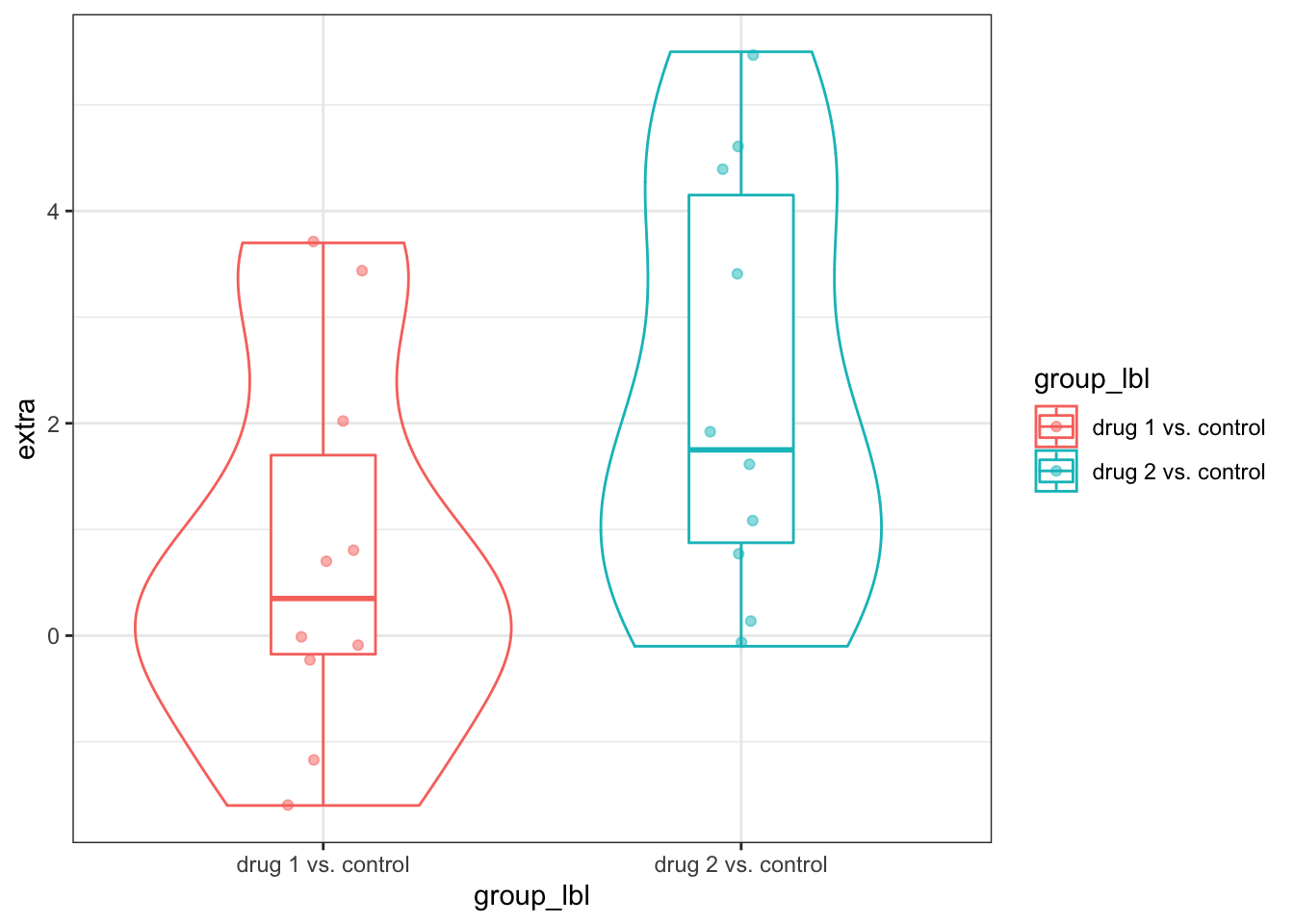## Test within-subjects effect using different approaches

### using t.test(paired = TRUE)

t.test(extra ~ group, data = sleep, paired = TRUE)
##
##  Paired t-test
##
## data:  extra by group
## t = -4.0621, df = 9, p-value = 0.002833
## alternative hypothesis: true difference in means is not equal to 0
## 95 percent confidence interval:
##  -2.4598858 -0.7001142
## sample estimates:
## mean of the differences
##                   -1.58

### Compute a difference score and test whether the mean difference = 0

# using dotproduct
t.test(with(sleep, cbind(extra[group == 1], extra[group == 2]) %*% c(-1, 1)))
##
##  One Sample t-test
##
## data:  with(sleep, cbind(extra[group == 1], extra[group == 2]) %*% c(-1,     1))
## t = 4.0621, df = 9, p-value = 0.002833
## alternative hypothesis: true mean is not equal to 0
## 95 percent confidence interval:
##  0.7001142 2.4598858
## sample estimates:
## mean of x
##      1.58
# subtracting group 1 from group 2
t.test(with(sleep, extra[group == 1] * -1 + extra[group == 2] * 1))
##
##  One Sample t-test
##
## data:  with(sleep, extra[group == 1] * -1 + extra[group == 2] * 1)
## t = 4.0621, df = 9, p-value = 0.002833
## alternative hypothesis: true mean is not equal to 0
## 95 percent confidence interval:
##  0.7001142 2.4598858
## sample estimates:
## mean of x
##      1.58

### Fit a linear mixed effects model

# create new contrast code called drug, which represents the drug effect on sleep increase
sleep$drug <- with(sleep, ifelse(group == 1, -0.5, 0.5)) # fit model lmer.fit1 <- lmer(extra ~ drug + (1 | ID), data = sleep, REML = TRUE) #### Diagnostic plots # residuals vs. fitted values plot(lmer.fit1, type = c("p", "smooth"))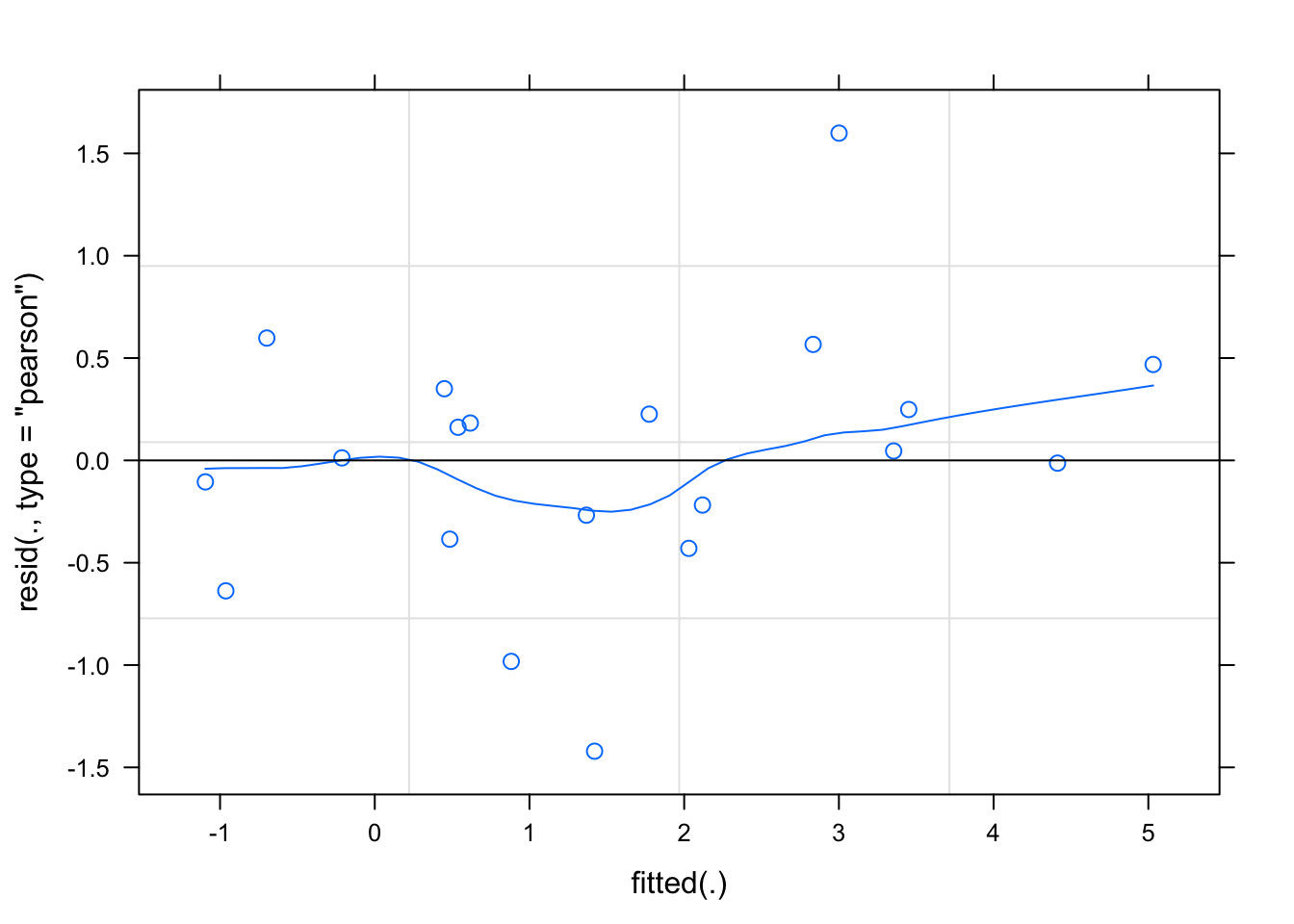# q-q plot of random effects qqmath(ranef(lmer.fit1, condVar = TRUE)) ##$ID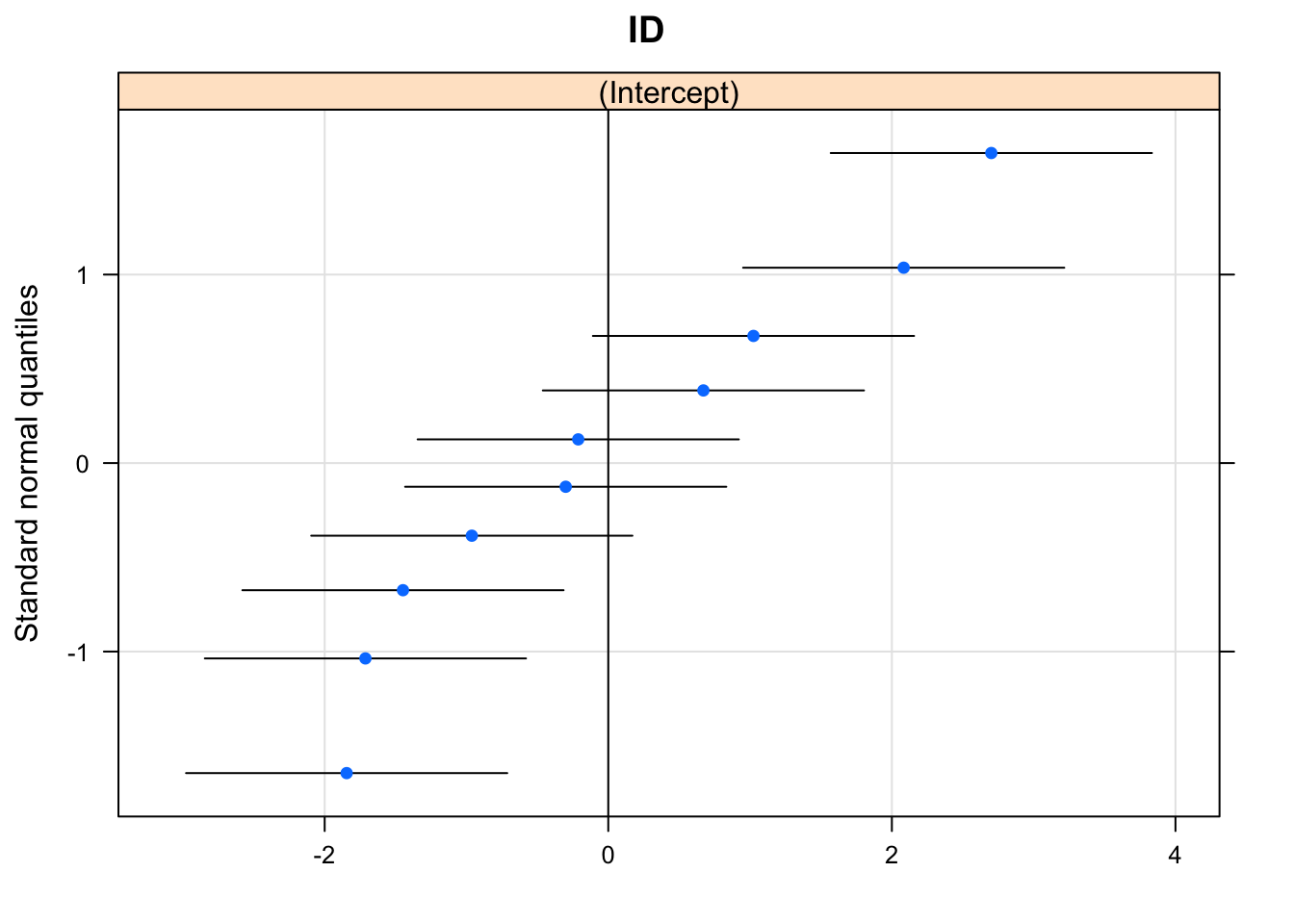# q-q plot of fixed effects
qqmath(lmer.fit1)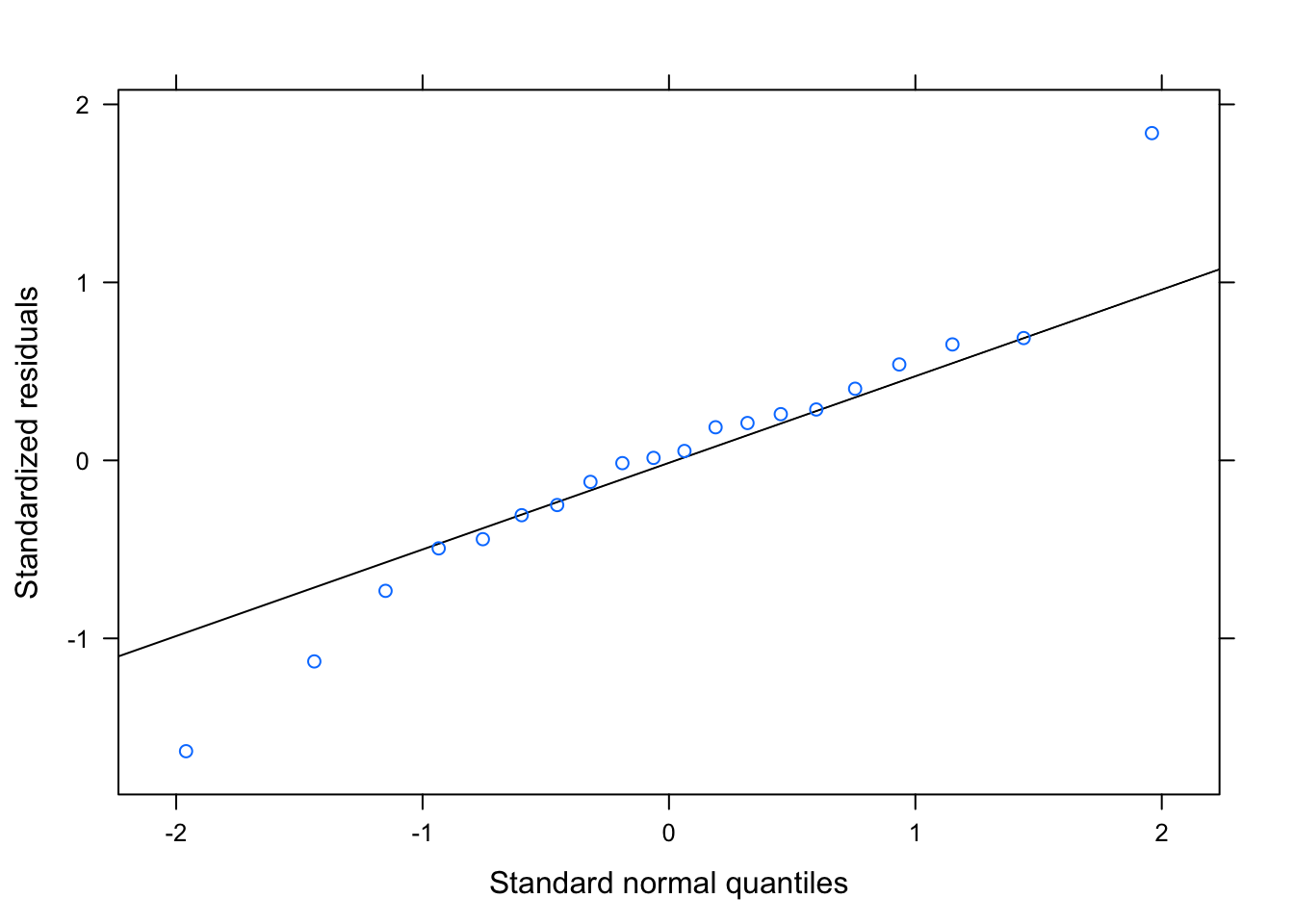# random effects estimates
dotplot(ranef(lmer.fit1, condVar = TRUE))
## $ID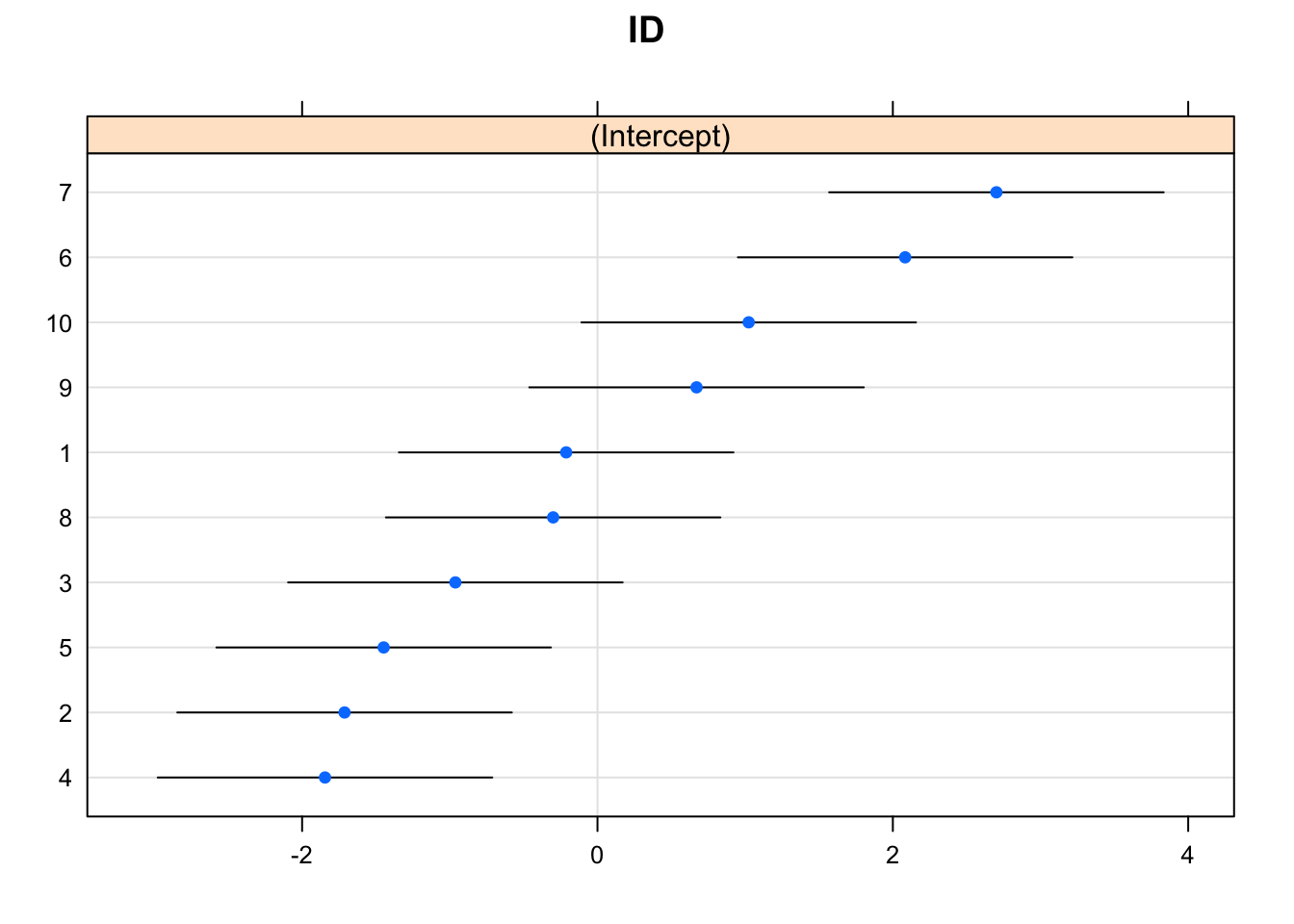#### Results e.g., variance components, fixed effect coefficients, residual summary, fit indices summary(lmer.fit1) ## Linear mixed model fit by REML. t-tests use Satterthwaite's method [ ## lmerModLmerTest] ## Formula: extra ~ drug + (1 | ID) ## Data: sleep ## ## REML criterion at convergence: 70 ## ## Scaled residuals: ## Min 1Q Median 3Q Max ## -1.63372 -0.34157 0.03346 0.31511 1.83859 ## ## Random effects: ## Groups Name Variance Std.Dev. ## ID (Intercept) 2.8483 1.6877 ## Residual 0.7564 0.8697 ## Number of obs: 20, groups: ID, 10 ## ## Fixed effects: ## Estimate Std. Error df t value Pr(>|t|) ## (Intercept) 1.540 0.568 9.000 2.711 0.02395 * ## drug 1.580 0.389 9.000 4.062 0.00283 ** ## --- ## Signif. codes: 0 '***' 0.001 '**' 0.01 '*' 0.05 '.' 0.1 ' ' 1 ## ## Correlation of Fixed Effects: ## (Intr) ## drug 0.000 # Here’s a more complex situation with an additional between-subjects factor ## Generate data # set random seed set.seed(8888) # id id <- factor(1:50) # two treatment conditions, each size = 25 treatment <- factor(rep(1:2, each = 25)) # labels in a separate column treatment_lbl <- ifelse(treatment == 1, "control", "treatment") # draws random numbers from true populations, depending on treatment value response1 <- rnorm(n = rep(25, 2)[treatment], mean = c(4, 5)[treatment], sd = c(1, 1)[treatment]) # response 2 should be correlated r = 0.5 with response 1, but the mean should be slightly higher response2 <- response1 * 0.5 + rnorm(n = 50, mean = c(1, 2)[treatment], sd = 1) # save and print data mydata <- tibble(id, treatment_lbl, treatment, response1, response2) kable(mydata) id treatment_lbl treatment response1 response2 1 control 1 4.195752 4.1745159 2 control 1 4.976012 1.7597310 3 control 1 3.963119 4.6346111 4 control 1 3.975639 3.3488885 5 control 1 4.022112 4.2694685 6 control 1 3.291423 1.8133618 7 control 1 3.074208 3.2192163 8 control 1 5.453050 3.8107214 9 control 1 3.843103 1.3888784 10 control 1 3.562954 2.4298635 11 control 1 3.482628 4.3645302 12 control 1 3.608857 3.4381481 13 control 1 3.360039 0.2610100 14 control 1 4.099164 5.8771612 15 control 1 4.088679 2.9806595 16 control 1 5.063440 2.4306743 17 control 1 4.361441 2.4265752 18 control 1 3.022724 0.3446552 19 control 1 4.879871 3.0962526 20 control 1 4.260023 2.5881955 21 control 1 3.201820 2.0445063 22 control 1 5.360563 3.6988670 23 control 1 3.087335 2.3934641 24 control 1 4.780342 3.9045912 25 control 1 5.244337 3.8680810 26 treatment 2 4.427888 4.4144632 27 treatment 2 3.382405 4.4673716 28 treatment 2 4.974487 2.7394246 29 treatment 2 3.258423 3.7922637 30 treatment 2 4.931010 4.3812514 31 treatment 2 5.809873 4.7746719 32 treatment 2 4.712183 5.0906109 33 treatment 2 5.443887 5.3443579 34 treatment 2 4.851525 2.5236780 35 treatment 2 6.003865 3.1215988 36 treatment 2 6.023490 5.8752659 37 treatment 2 6.516993 7.1395760 38 treatment 2 6.122140 5.2266728 39 treatment 2 5.639430 4.5648023 40 treatment 2 6.431992 5.2331465 41 treatment 2 3.166982 5.1602065 42 treatment 2 5.618815 5.5389033 43 treatment 2 4.846700 4.6647061 44 treatment 2 3.910692 5.3452577 45 treatment 2 5.993828 4.8716862 46 treatment 2 3.465042 2.9443906 47 treatment 2 5.742368 4.3487534 48 treatment 2 3.460245 4.3271617 49 treatment 2 3.854740 4.8651375 50 treatment 2 4.719107 6.0689389 # restructure lmydata <- gather(mydata, key = sequence_lbl, value = response, response1:response2) %>% mutate(sequence = ifelse(sequence_lbl == "response1", -0.5, 0.5), treatment = ifelse(treatment == 1, -0.5, 0.5)) ## Visualize relationships It’s always a good idea to look at your data. Check some assumptions. ggplot(lmydata, mapping = aes(x = sequence_lbl, y = response, color = treatment_lbl)) + geom_violin(position = position_dodge(width = 0.90), alpha = 0) + geom_boxplot(outlier.color = NA, width = 0.25, position = position_dodge(width = 0.90)) + geom_point(position = position_jitterdodge(dodge.width = 0.90, jitter.width = 0.10), alpha = 0.40) + theme_bw()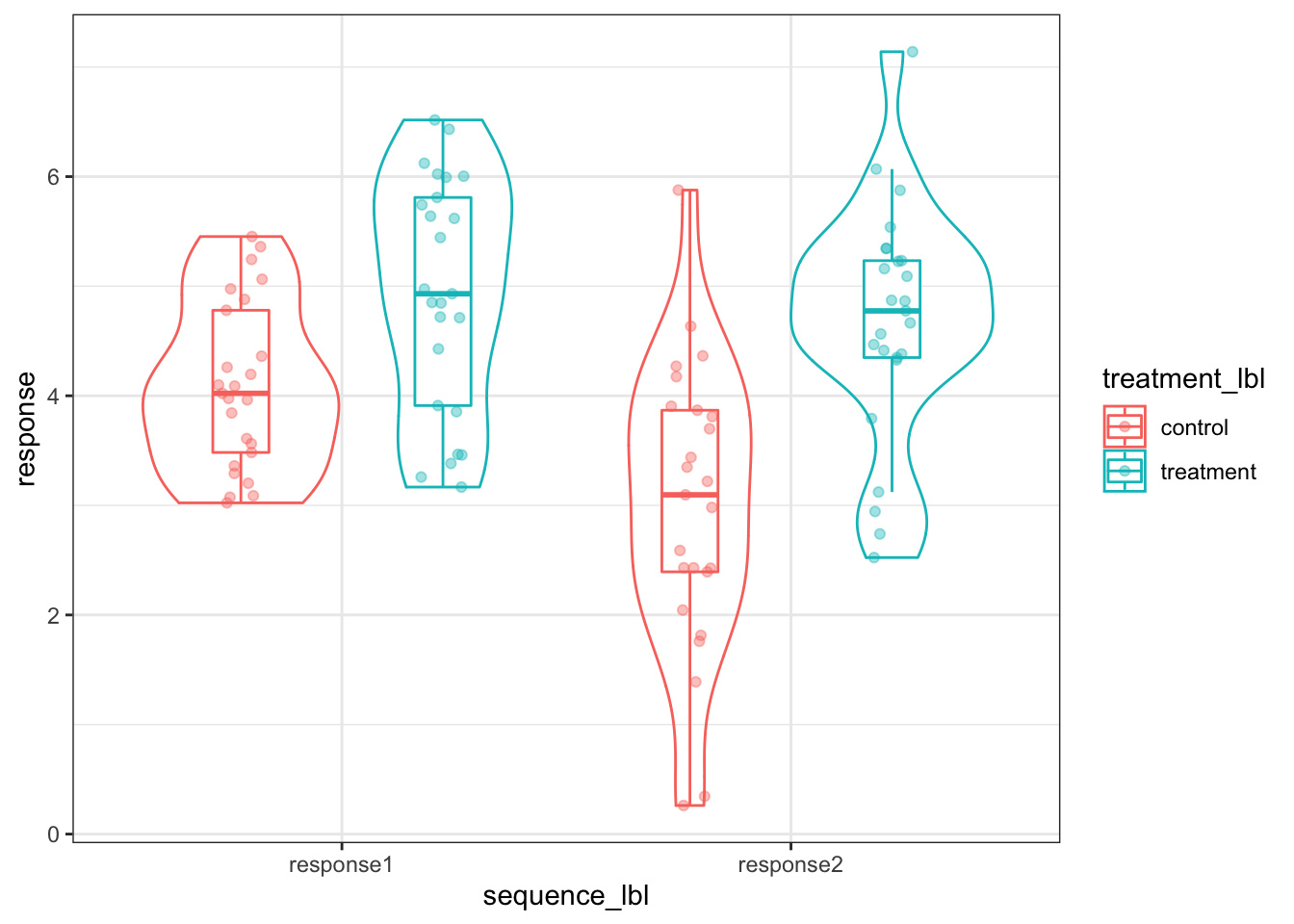## Test effects using different approaches ### Test average treatment effect (between-subjects) # create new columns that averages the responses mydata$response_avg <- with(mydata, (response1 + response2) / 2)

# using t.test
t.test(response_avg ~ treatment_lbl, data = mydata)
##
##  Welch Two Sample t-test
##
## data:  response_avg by treatment_lbl
## t = -5.1627, df = 47.999, p-value = 4.622e-06
## alternative hypothesis: true difference in means is not equal to 0
## 95 percent confidence interval:
##  -1.7592474 -0.7730385
## sample estimates:
##   mean in group control mean in group treatment
##                3.536505                4.802648
# using oneway function
oneway(dv = mydata$response_avg, group = mydata$treatment_lbl, contrast = list(treatment_lbl = c(-1, 1)), alpha = 0.05)
##        contrast   equal_var      est       se        t       df
## 1 treatment_lbl     Assumed 1.266143 0.245248 5.162703 48.00000
## 2 treatment_lbl Not Assumed 1.266143 0.245248 5.162703 47.99921
##              p       lwr      upr
## 1 4.621802e-06 0.7730387 1.759247
## 2 4.621969e-06 0.7730385 1.759247
# using oneway function and dotproduct
oneway(dv = as.numeric(as.matrix(mydata[, c("response1", "response2")]) %*% c(1, 1) / 2), group = mydata$treatment_lbl, contrast = list(treatment_lbl = c(-1, 1)), alpha = 0.05) ## contrast equal_var est se t df ## 1 treatment_lbl Assumed 1.266143 0.245248 5.162703 48.00000 ## 2 treatment_lbl Not Assumed 1.266143 0.245248 5.162703 47.99921 ## p lwr upr ## 1 4.621802e-06 0.7730387 1.759247 ## 2 4.621969e-06 0.7730385 1.759247 ### Test sequence effect (within-subjects) via multivariate approach Average sequence effect will differ depending on whether other terms are included in the model # create new columns that reflects difference between two response mydata$response_diff <- with(mydata, -response1 + response2)

# using t.test on difference score, compare to mean = 0
with(mydata, t.test(response_diff))
##
##  One Sample t-test
##
## data:  response_diff
## t = -3.6779, df = 49, p-value = 0.000584
## alternative hypothesis: true mean is not equal to 0
## 95 percent confidence interval:
##  -1.0569850 -0.3100477
## sample estimates:
##  mean of x
## -0.6835164
# using paired t.test
t.test(response ~ sequence_lbl, data = lmydata, paired = TRUE)
##
##  Paired t-test
##
## data:  response by sequence_lbl
## t = 3.6779, df = 49, p-value = 0.000584
## alternative hypothesis: true difference in means is not equal to 0
## 95 percent confidence interval:
##  0.3100477 1.0569850
## sample estimates:
## mean of the differences
##               0.6835164
# using oneway function
oneway(dv = mydata$response_diff, group = mydata$treatment_lbl, contrast = list(treatment_lbl = c(1, 1) / 2), alpha = 0.05)
##        contrast   equal_var        est        se         t       df
## 1 treatment_lbl     Assumed -0.6835164 0.1775092 -3.850597 48.00000
## 2 treatment_lbl Not Assumed -0.6835164 0.1775092 -3.850597 47.97627
##              p       lwr        upr
## 1 0.0003484712 -1.040423 -0.3266102
## 2 0.0003486282 -1.040427 -0.3266056
# using oneway function and dotproduct
oneway(dv = as.numeric(as.matrix(mydata[, c("response1", "response2")]) %*% c(-1, 1)), group = mydata$treatment_lbl, contrast = list(treatment_lbl = c(1, 1) / 2), alpha = 0.05) ## contrast equal_var est se t df ## 1 treatment_lbl Assumed -0.6835164 0.1775092 -3.850597 48.00000 ## 2 treatment_lbl Not Assumed -0.6835164 0.1775092 -3.850597 47.97627 ## p lwr upr ## 1 0.0003484712 -1.040423 -0.3266102 ## 2 0.0003486282 -1.040427 -0.3266056 ### Test treatment by sequence effect via multivariate approach # using independent samples welch t.test t.test(response_diff ~ treatment_lbl, data = mydata) ## ## Welch Two Sample t-test ## ## data: response_diff by treatment_lbl ## t = -2.3895, df = 47.976, p-value = 0.02085 ## alternative hypothesis: true difference in means is not equal to 0 ## 95 percent confidence interval: ## -1.5621492 -0.1345063 ## sample estimates: ## mean in group control mean in group treatment ## -1.1076802 -0.2593525 # using oneway function oneway(dv = mydata$response_diff, group = mydata$treatment_lbl, contrast = list(treatment_lbl = c(-1, 1)), alpha = 0.05) ## contrast equal_var est se t df ## 1 treatment_lbl Assumed 0.8483278 0.3550184 2.389532 48.00000 ## 2 treatment_lbl Not Assumed 0.8483278 0.3550184 2.389532 47.97627 ## p lwr upr ## 1 0.02084609 0.1345154 1.562140 ## 2 0.02084812 0.1345063 1.562149 # using oneway function and dotproduct oneway(dv = as.numeric(as.matrix(mydata[, c("response1", "response2")]) %*% c(-1, 1)), group = mydata$treatment_lbl, contrast = list(treatment_lbl = c(-1, 1)), alpha = 0.05)
##        contrast   equal_var       est        se        t       df
## 1 treatment_lbl     Assumed 0.8483278 0.3550184 2.389532 48.00000
## 2 treatment_lbl Not Assumed 0.8483278 0.3550184 2.389532 47.97627
##            p       lwr      upr
## 1 0.02084609 0.1345154 1.562140
## 2 0.02084812 0.1345063 1.562149

### Test treatment by sequence effect via linear mixed effects model

#### Fit a linear mixed effects model

lmer.fit2 <- lmer(response ~ treatment * sequence + (1 + sequence | id), data = lmydata, REML = TRUE, control = lmerControl(check.nobs.vs.nlev = "ignore", check.nobs.vs.nRE = "ignore"))
## Warning in checkConv(attr(opt, "derivs"), opt$par, ctrl = ## control$checkConv, : unable to evaluate scaled gradient
## Warning in checkConv(attr(opt, "derivs"), opt$par, ctrl = ## control$checkConv, : Model failed to converge: degenerate Hessian with 1
## negative eigenvalues
## Warning: Model failed to converge with 1 negative eigenvalue: -1.9e-03

#### Diagnostic plots

# residuals vs. fitted values
plot(lmer.fit1, type = c("p", "smooth"))# q-q plot of random effects
qqmath(ranef(lmer.fit1, condVar = TRUE))
## $ID# q-q plot of fixed effects qqmath(lmer.fit1)# random effects estimates dotplot(ranef(lmer.fit1, condVar = TRUE)) ##$ID#### Results

e.g., variance components, fixed effect coefficients, residual summary, fit indices

summary(lmer.fit2)
## Linear mixed model fit by REML. t-tests use Satterthwaite's method [
## lmerModLmerTest]
## Formula: response ~ treatment * sequence + (1 + sequence | id)
##    Data: lmydata
## Control:
## lmerControl(check.nobs.vs.nlev = "ignore", check.nobs.vs.nRE = "ignore")
##
## REML criterion at convergence: 290.1
##
## Scaled residuals:
##      Min       1Q   Median       3Q      Max
## -1.71150 -0.45806 -0.00089  0.43872  1.58043
##
## Random effects:
##  Groups   Name        Variance Std.Dev. Corr
##  id       (Intercept) 0.5068   0.7119
##           sequence    0.5947   0.7711   0.52
##  Residual             0.4903   0.7002
## Number of obs: 100, groups:  id, 50
##
## Fixed effects:
##                    Estimate Std. Error      df t value Pr(>|t|)
## (Intercept)          4.1696     0.1226 47.9928  34.001  < 2e-16 ***
## treatment            1.2661     0.2453 47.9928   5.162 4.63e-06 ***
## sequence            -0.6835     0.1775 48.0030  -3.851 0.000348 ***
## treatment:sequence   0.8483     0.3550 48.0030   2.390 0.020839 *
## ---
## Signif. codes:  0 '***' 0.001 '**' 0.01 '*' 0.05 '.' 0.1 ' ' 1
##
## Correlation of Fixed Effects:
##             (Intr) trtmnt sequnc
## treatment   0.000
## sequence    0.261  0.000
## trtmnt:sqnc 0.000  0.261  0.000
## convergence code: 0
## unable to evaluate scaled gradient
## Model failed to converge: degenerate  Hessian with 1 negative eigenvalues

# Here’s an example from the Maxwell, Dalaney, and Kelley (2017) textbook

From help("C14T8"): “For the hypothetical data contained in Table 14.8, a perceptual psychologist is interested in age differences (”young" and “old”) in reaction time on a perceptual task. In addition, the psychologist is also interested in the effect of angle (zero degrees off center and eight degrees off center). The question of interest is to see if there are is a main effect of age, a main effect of angle, and an interaction between the two. Table 14.8 presents the same data that we analyzed in chapter 12 for 10 young participants and 10 old participants, except that for the moment we are only analyzing data from the 0 degree and 8 degree conditions of the angle factor."

data("C14T8")

# there is a slight difference between the textbook data and the data available in the package -- I fix that here
C14T8 <- C14T8 %>%
mutate(subj = factor(1:20),
Angle4 = c(510, 480, 630, 660, 660, 450, 600, 660, 660, 540, 570, 720, 540, 660, 570, 780, 690, 570, 750, 690),
age = recode(Group, "1" = -0.5, "2" = 0.5),
age_lbl = recode(Group, "1" = "young", "2" = "old")) %>%
select(subj, Group, age, age_lbl, Angle0, Angle4, Angle8)

# print
kable(C14T8)
subj Group age age_lbl Angle0 Angle4 Angle8
1 1 -0.5 young 450 510 630
2 1 -0.5 young 390 480 540
3 1 -0.5 young 570 630 660
4 1 -0.5 young 450 660 720
5 1 -0.5 young 510 660 630
6 1 -0.5 young 360 450 450
7 1 -0.5 young 510 600 720
8 1 -0.5 young 510 660 780
9 1 -0.5 young 510 660 660
10 1 -0.5 young 510 540 660
11 2 0.5 old 420 570 690
12 2 0.5 old 600 720 810
13 2 0.5 old 450 540 690
14 2 0.5 old 630 660 780
15 2 0.5 old 420 570 780
16 2 0.5 old 600 780 870
17 2 0.5 old 630 690 870
18 2 0.5 old 480 570 720
19 2 0.5 old 690 750 900
20 2 0.5 old 510 690 810

### Restructure data

lC14T8 <- C14T8 %>%
gather(key = angle, value = rt, Angle0, Angle4, Angle8) %>%
mutate(angle_num = str_sub(angle, start = nchar(angle), end = nchar(angle)) %>% as.numeric(),
angle_lbl = recode(angle_num, "0" = "0 degrees", "4" = "4 degrees", "8" = "8 degrees"),
angle_linear = recode(angle_num, "0" = -0.5, "4" = 0, "8" = 0.5),
angle_quadratic = recode(angle_num, "0" = 0.5, "4" = -1, "8" = 0.5))

### Visualize relationships

It’s always a good idea to look at your data. Check some assumptions.

ggplot(lC14T8, mapping = aes(x = angle_lbl, y = rt, color = age_lbl)) +
geom_violin(position = position_dodge(width = 0.90), alpha = 0) +
geom_boxplot(outlier.color = NA, width = 0.25, position = position_dodge(width = 0.90)) +
geom_point(position = position_jitterdodge(dodge.width = 0.90, jitter.width = 0.10), alpha = 0.40) +
theme_bw()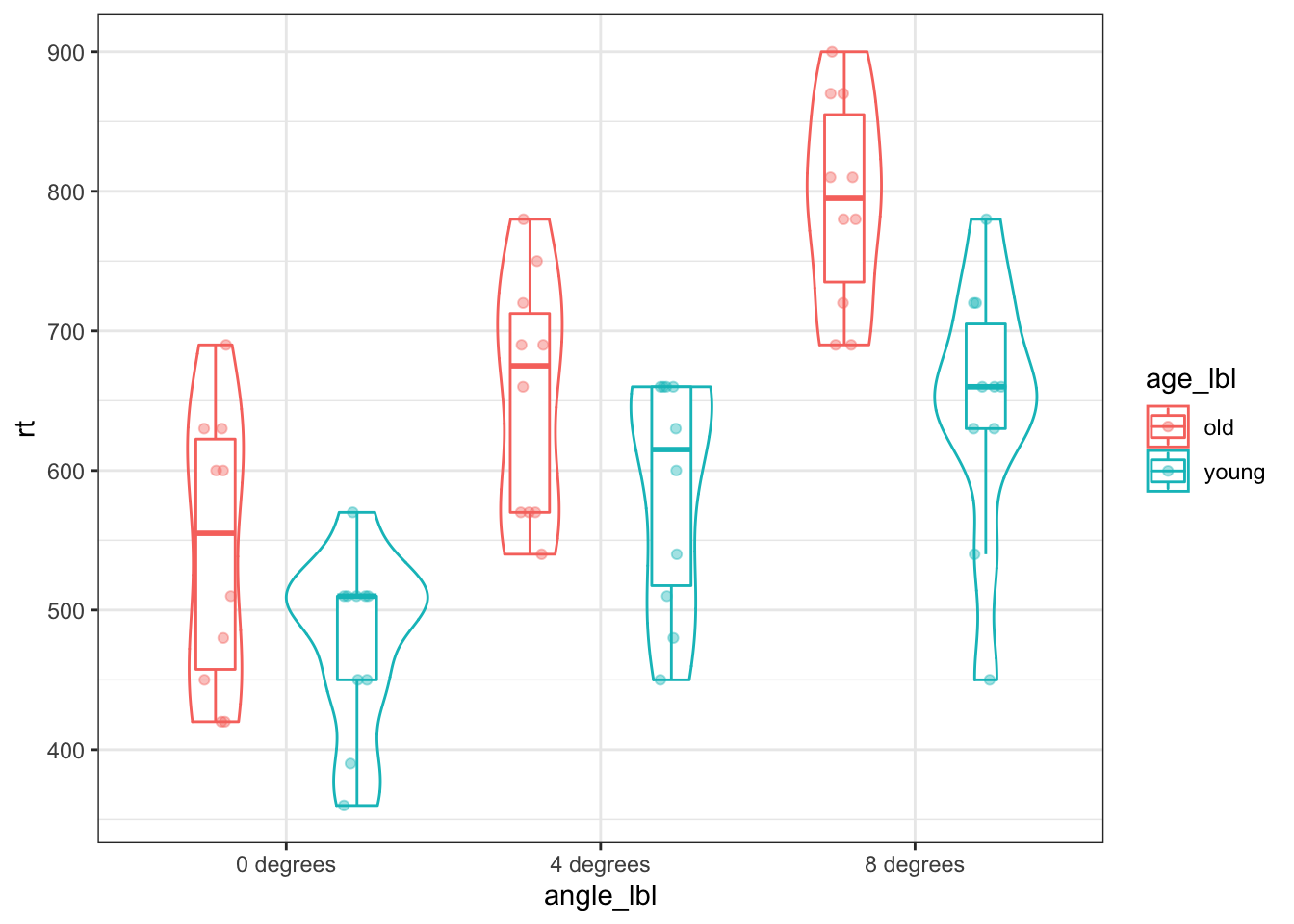## Test effects using different approaches

### Test age effect (between-subjects) via multivariate approach

c(1, 2, 3) %*% c(-1, 0, 1) means take the dot product of the matrix c(1, 2, 3) and the matrix c(-1, 0, 1), and this means multiply 1 by -1, 2 by 0, and 3 by 1, and then add up those products

oneway(dv = as.numeric(as.matrix(C14T8[, c("Angle0", "Angle4", "Angle8")]) %*% (c(1, 1, 1) / 3)), group = C14T8$Group, contrast = list(age = c(-1, 1))) ## contrast equal_var est se t df p lwr ## 1 age Assumed 94 34.84888 2.697361 18.00000 0.01473401 20.78522 ## 2 age Not Assumed 94 34.84888 2.697361 17.78843 0.01483957 20.72275 ## upr ## 1 167.2148 ## 2 167.2772 ### Test angle linear effect (within-subjects) c(1, 2, 3) %*% c(-1, 0, 1) means tke the dot product of the matrix c(1, 2, 3) and the matrix c(-1, 0, 1), and this this means multiply 1 by -1, 2 by 0, and 3 by 1, and then add up those products oneway(dv = as.numeric(as.matrix(C14T8[, c("Angle0", "Angle4", "Angle8")]) %*% c(-1, 0, 1)), group = C14T8$Group, contrast = list(age = c(1, 1) / 2))
##   contrast   equal_var   est       se       t       df            p
## 1      age     Assumed 208.5 13.64734 15.2777 18.00000 9.479528e-12
## 2      age Not Assumed 208.5 13.64734 15.2777 17.66239 1.277400e-11
##        lwr      upr
## 1 179.8280 237.1720
## 2 179.7887 237.2113

### Test angle quadratic effect (within-subjects)

c(1, 2, 3) %*% c(-1, 0, 1) means tke the dot product of the matrix c(1, 2, 3) and the matrix c(-1, 0, 1), and this this means multiply 1 by -1, 2 by 0, and 3 by 1, and then add up those products

oneway(dv = as.numeric(as.matrix(C14T8[, c("Angle0", "Angle4", "Angle8")]) %*% c(1, -2, 1) / 3), group = C14T8$Group, contrast = list(age = c(1, 1) / 2)) ## contrast equal_var est se t df p ## 1 age Assumed -3.5 6.220486 -0.562657 18.00000 0.5806094 ## 2 age Not Assumed -3.5 6.220486 -0.562657 17.01589 0.5810066 ## lwr upr ## 1 -16.56876 9.568756 ## 2 -16.62314 9.623144 ### Test angle linear effect x age effect (mixed) c(1, 2, 3) %*% c(-1, 0, 1) means tke the dot product of the matrix c(1, 2, 3) and the matrix c(-1, 0, 1), and this this means multiply 1 by -1, 2 by 0, and 3 by 1, and then add up those products oneway(dv = as.numeric(as.matrix(C14T8[, c("Angle0", "Angle4", "Angle8")]) %*% c(-1, 0, 1)), group = C14T8$Group, contrast = list(age = c(-1, 1)))
##   contrast   equal_var est       se       t       df           p      lwr
## 1      age     Assumed  81 27.29469 2.96761 18.00000 0.008245640 23.65599
## 2      age Not Assumed  81 27.29469 2.96761 17.66239 0.008369684 23.57732
##        upr
## 1 138.3440
## 2 138.4227

### Test angle quadratic effect x age effect (mixed)

c(1, 2, 3) %*% c(-1, 0, 1) means tke the dot product of the matrix c(1, 2, 3) and the matrix c(-1, 0, 1), and this this means multiply 1 by -1, 2 by 0, and 3 by 1, and then add up those products

oneway(dv = as.numeric(as.matrix(C14T8[, c("Angle0", "Angle4", "Angle8")]) %*% c(1, -2, 1) / 3), group = C14T8$Group, contrast = list(age = c(-1, 1))) ## contrast equal_var est se t df p lwr ## 1 age Assumed 25 12.44097 2.009489 18.00000 0.05972078 -1.137512 ## 2 age Not Assumed 25 12.44097 2.009489 17.01589 0.06061833 -1.246289 ## upr ## 1 51.13751 ## 2 51.24629 ### Test angle by age effects via linear mixed effects model #### Fit a linear mixed effects model lmer.fit3 <- lmer(rt ~ (angle_linear + angle_quadratic) * age + (1 + angle_linear + angle_quadratic | subj), data = lC14T8, REML = TRUE, control = lmerControl(check.nobs.vs.nRE = "ignore", check.nobs.vs.nlev = "ignore")) #### Diagnostic plots # residuals vs. fitted values plot(lmer.fit3, type = c("p", "smooth"))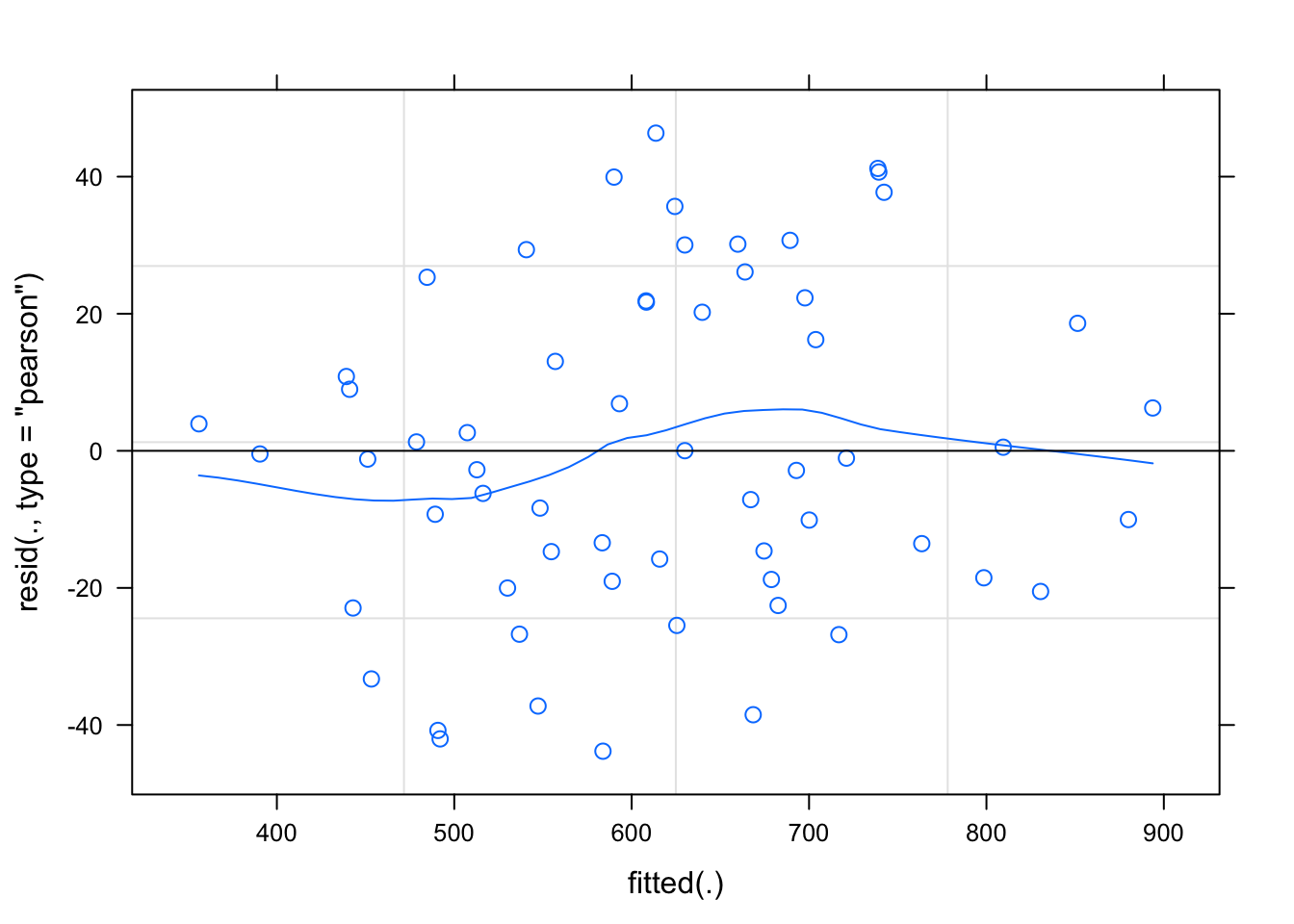# q-q plot of random effects qqmath(ranef(lmer.fit3, condVar = TRUE)) ##$subj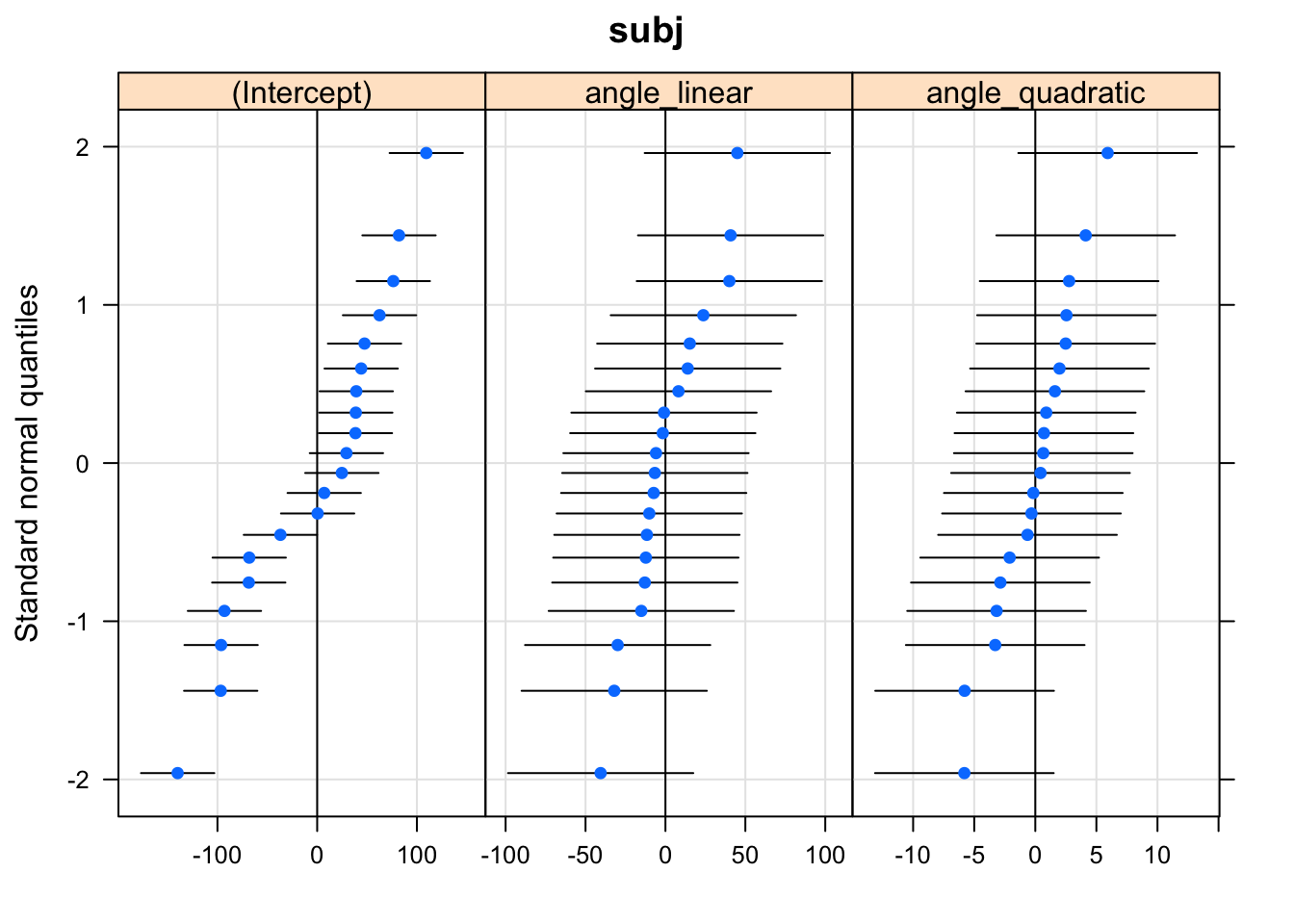# q-q plot of fixed effects
qqmath(lmer.fit3)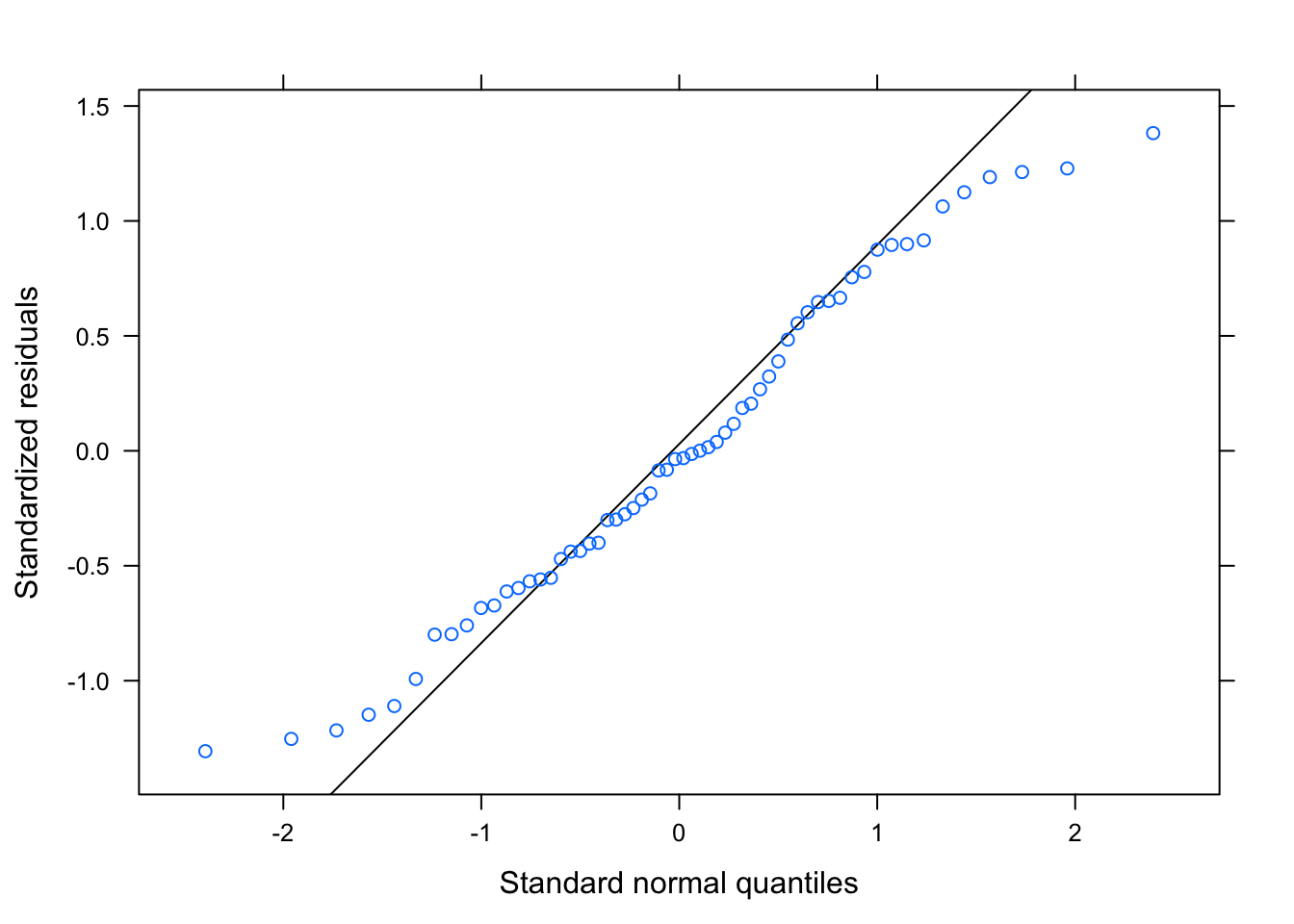# random effects estimates
dotplot(ranef(lmer.fit3, condVar = TRUE))
## \$subj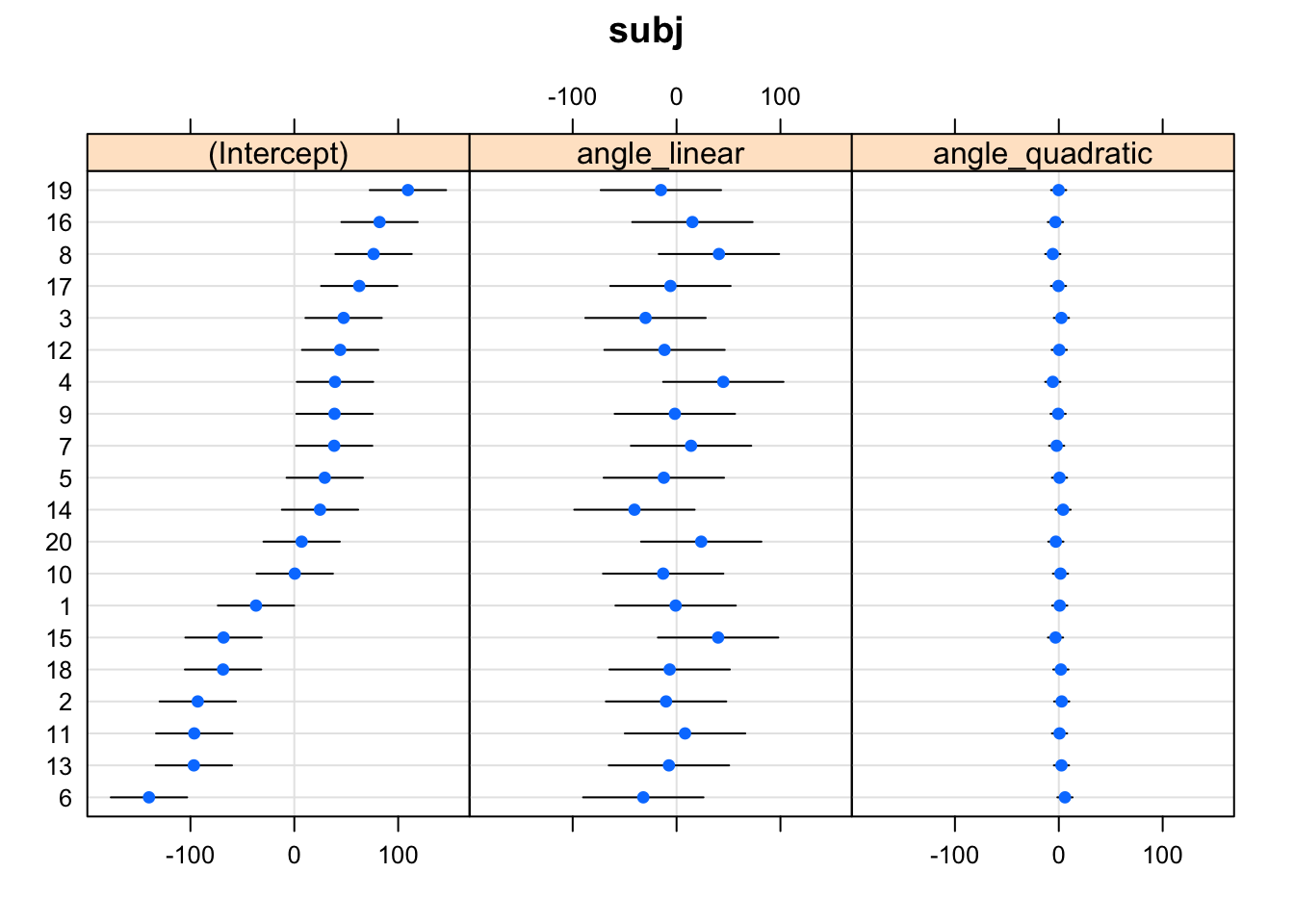#### Results

e.g., variance components, fixed effect coefficients, residual summary, fit indices

summary(lmer.fit3)
## Linear mixed model fit by REML. t-tests use Satterthwaite's method [
## lmerModLmerTest]
## Formula:
## rt ~ (angle_linear + angle_quadratic) * age + (1 + angle_linear +
##    Data: lC14T8
## Control:
## lmerControl(check.nobs.vs.nRE = "ignore", check.nobs.vs.nlev = "ignore")
##
## REML criterion at convergence: 607.5
##
## Scaled residuals:
##      Min       1Q   Median       3Q      Max
## -1.30665 -0.55417 -0.03389  0.61377  1.38200
##
## Random effects:
##  Groups   Name            Variance Std.Dev. Corr
##  subj     (Intercept)     5696.69  75.476
##           angle_linear    1475.59  38.413    0.09
##           angle_quadratic   24.03   4.902   -0.35 -0.89
##  Residual                 1124.79  33.538
## Number of obs: 60, groups:  subj, 20
##
## Fixed effects:
##                     Estimate Std. Error     df t value Pr(>|t|)
## (Intercept)           616.00      17.42  18.00  35.354  < 2e-16 ***
## angle_linear          208.50      13.65  18.00  15.277 9.48e-12 ***
## angle_quadratic        -3.50       6.22  18.00  -0.563  0.58061
## age                    94.00      34.85  18.00   2.697  0.01473 *
## angle_linear:age       81.00      27.30  18.00   2.968  0.00825 **
## angle_quadratic:age    25.00      12.44  18.00   2.009  0.05972 .
## ---
## Signif. codes:  0 '***' 0.001 '**' 0.01 '*' 0.05 '.' 0.1 ' ' 1
##
## Correlation of Fixed Effects:
##             (Intr) angl_l angl_q age    angl_l:
## angle_liner  0.055
## angle_qdrtc -0.060 -0.099
## age          0.000  0.000  0.000
## angle_lnr:g  0.000  0.000  0.000  0.055
## angl_qdrtc:  0.000  0.000  0.000 -0.060 -0.099

# Export data for use in SPSS

write_csv(lC14T8, path = "lC14T8.csv")

# Resources

• Boik, R. J. (1981). A priori tests in repeated measures designs: Effects of nonsphericity. Psychometrika, 46(3), 241-255.
• Maxwell, S. E., Delaney, H. D., & Kelley, K. (2018). Designing experiments and analyzing data: A model comparison perspective (3rd ed.). Routledge.
• DESIGNING EXPERIMENTS AND ANALYZING DATA includes computer code for analyses from their book, Shiny apps, and more.

# General word of caution

Above, I listed resources prepared by experts on these and related topics. Although I generally do my best to write accurate posts, don’t assume my posts are 100% accurate or that they apply to your data or research questions. Trust statistics and methodology experts, not blog posts.##### Nicholas M. Michalak
###### Ph.D. Candidate, Social Psychology

I study how both modern and evolutionarily-relevant threats affect how people perceive themselves and others.